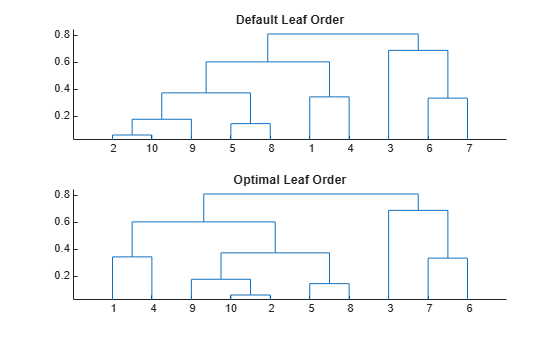optimalleaforder

Optimal leaf ordering for hierarchical clustering

Description

example

leafOrder = optimalleaforder(tree,D) returns an optimal leaf ordering for the hierarchical binary cluster tree, tree, using the distances, D. An optimal leaf ordering of a binary tree maximizes the sum of the similarities between adjacent leaves by flipping tree branches without dividing the clusters.

leafOrder = optimalleaforder(tree,D,Name,Value) returns the optimal leaf ordering using one or more name-value pair arguments.

Examples

collapse all

Create a hierarchical binary cluster tree using linkage. Then, compare the dendrogram plot with the default ordering to a dendrogram with an optimal leaf ordering.

Generate sample data.

rng('default') % For reproducibility
X = rand(10,2);

Create a distance vector and a hierarchical binary clustering tree. Use the distances and clustering tree to determine an optimal leaf order.

D = pdist(X);
leafOrder = optimalleaforder(tree,D);

Plot the dendrogram with the default ordering and the dendrogram with the optimal leaf ordering.

figure()
subplot(2,1,1)
dendrogram(tree)
title('Default Leaf Order')

subplot(2,1,2)
dendrogram(tree,'reorder',leafOrder)
title('Optimal Leaf Order')The order of the leaves in the bottom figure corresponds to the elements in leafOrder.

leafOrder
leafOrder = 1×10

1     4     9    10     2     5     8     3     7     6

Generate sample data.

rng('default') % For reproducibility
X = rand(10,2);

Create a distance vector and a hierarchical binary clustering tree.

D = pdist(X);

Use the inverse distance similarity transformation to determine an optimal leaf order.

leafOrder = optimalleaforder(tree,D,'Transformation','inverse')
leafOrder = 1×10

1     4     9    10     2     5     8     3     7     6

Input Arguments

collapse all

Hierarchical binary cluster tree, specified as an (M – 1)-by-3 matrix that you generate using linkage, where M is the number of leaves.

Distances for determining similarities between leaves, specified as a matrix or vector of distances. For example, you can generate distances using pdist.

Name-Value Arguments

Specify optional comma-separated pairs of Name,Value arguments. Name is the argument name and Value is the corresponding value. Name must appear inside quotes. You can specify several name and value pair arguments in any order as Name1,Value1,...,NameN,ValueN.

Example: 'Criteria','group','Transformation','inverse' specifies that the sum of similarities be maximized between every leaf and all other leaves in adjacent clusters, using an inverse similarity transformation.

Optimization criterion for determining an optimal leaf ordering, specified as the comma-separated pair consisting of 'criteria' and one of these values:

 'adjacent' Maximize the sum of similarities between adjacent leaves. 'group' Maximize the sum of similarities between every leaf and all other leaves in the adjacent clusters at the same level of the dendrogram.

Example: 'Criteria','group'

Method for transforming distances to similarities, specified as the comma-separated pair consisting of 'Transformation' and one of 'linear', 'inverse', or a function handle.

Let di,j and Simi,j denote the distance and similarity between leaves i and j, respectively. The included similarity transformations are:

 'linear' Simi,j = maxi,j (di,j ) – di,j 'inverse' Simi,j = 1/di,j

To use a custom transformation function, specify a handle to a function that accepts a matrix of distances, D, and returns a matrix of similarities, S. The function should be monotonic decreasing in the range of distance values. S must have the same size as D, with S(i,j) being the similarity computed based on D(i,j).

Example: 'Transformation',@myTransform

Output Arguments

collapse all

Optimal leaf order, returned as a length-M vector, where M is the number of leaves. leafOrder is a permutation of the vector 1:M, giving an optimal leaf ordering based on the specified distances and similarity transformation.

 Bar-Joseph, Z., Gifford, D.K., and Jaakkola, T.S. (2001). "Fast optimal leaf ordering for hierarchical clustering." Bioinformatics Vol. 17, Suppl 1:S22–9. PMID: 11472989.